Дана: 0

Барлыгы: 0,00

0

# Cube puzzle

### Cube puzzle

Building cubes shown in several views from the available unit cubes aids spatial vision and other skills.

Математика

Этикеткалар

cube puzzle, 3D puzzle, куб, view, front view, бейнелеу, view from the back, ойын, solid geometry, математика

Байланысты экстралар

### Байланысты экстралар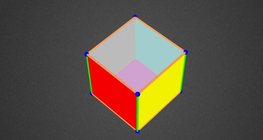#### Colouring a cube

Colouring the vertices, edges and faces of a given cube according to the criteria...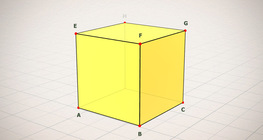#### Cube (exercises)

Edges, diagonals and faces of a cube can be identified by its vertices.#### Cube of cubes

An exercise about the regular hexahedron built from unit cubes to help deepen your...#### Cube sections (exercise)

Examining solids formed by the intersection of a cube and a plane.#### Net of a cube (exercises)

Not all nets consisting of 6 congruent squares are foldable into cubes.#### Building shapes (multi colour)

Build 3D shapes from unit cubes with the help of several views.#### Building shapes (one colour)

Build 3D shapes from unit cubes with the help of several views.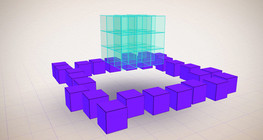#### Constructing shapes (3D)

Building 3D shapes into a given cube mesh with the help of several views.#### Cuboid

A cuboid is a polyhedron with six rectangular faces.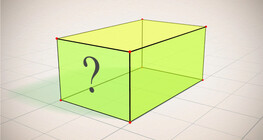#### Cuboid (exercises)

Edges, diagonals and faces of a cuboid can be identified by its vertices.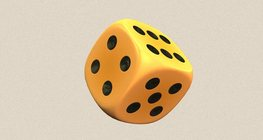#### Dice

Regular dice can be used for solving statistical and probability exercises.#### Grouping of cuboids

This animation demonstrates various types of cuboids through everyday objects.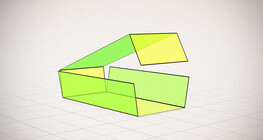#### Nets of a cuboid (exercises)

This animation demonstrates the different nets of a cuboid and includes a game.#### Shape games

Find views and nets of three-dimensional shapes.#### Volume and surface area (exercise)

An exercise about the volume and surface area of solids generated from a ´base cube´.